### Machine Learning – Introduction

Today’s Artificial Intelligence (AI) has far surpassed the hype of blockchain and quantum computing. This is due to the fact that huge computing resources are easily available to the common man. The developers now take advantage of this in creating new Machine Learning models and to re-train the existing models for better performance and results. The easy availability of High Performance Computing (HPC) has resulted in a sudden increased demand for IT professionals having Machine Learning skills.

In this tutorial, you will learn in detail about −

What is the crux of machine learning?

• What are the different types in machine learning?
• What are the different algorithms available for developing machine learning models?
• What tools are available for developing these models?
• What are the programming language choices?
• What platforms support development and deployment of Machine Learning applications?
• What IDEs (Integrated Development Environment) are available?

### Machine Learning – What Today’s AI Can Do?

When you tag a face in a Facebook photo, it is AI that is running behind the scenes and identifying faces in a picture. Face tagging is now omnipresent in several applications that display pictures with human faces. Why just human faces? There are several applications that detect objects such as cats, dogs, bottles, cars, etc. We have autonomous cars running on our roads that detect objects in real time to steer the car. When you travel, you use Google Directions to learn the real-time traffic situations and follow the best path suggested by Google at that point of time. This is yet another implementation of object detection technique in real time.

Let us consider the example of Google Translate application that we typically use while visiting foreign countries. Google’s online translator app on your mobile helps you communicate with the local people speaking a language that is foreign to you.

There are several applications of AI that we use practically today. In fact, each one of us use AI in many parts of our lives, even without our knowledge. Today’s AI can perform extremely complex jobs with a great accuracy and speed. Let us discuss an example of complex task to understand what capabilities are expected in an AI application that you would be developing today for your clients.

#### Example

We all use Google Directions during our trip anywhere in the city for a daily commute or even for inter-city travels. Google Directions application suggests the fastest path to our destination at that time instance. When we follow this path, we have observed that Google is almost 100% right in its suggestions and we save our valuable time on the trip.

You can imagine the complexity involved in developing this kind of application considering that there are multiple paths to your destination and the application has to judge the traffic situation in every possible path to give you a travel time estimate for each such path. Besides, consider the fact that Google Directions covers the entire globe. Undoubtedly, lots of AI and Machine Learning techniques are in-use under the hoods of such applications.

Considering the continuous demand for the development of such applications, you will now appreciate why there is a sudden demand for IT professionals with AI skills.

In our next chapter, we will learn what it takes to develop AI programs.

### Machine Learning – Traditional AI

The journey of AI began in the 1950’s when the computing power was a fraction of what it is today. AI started out with the predictions made by the machine in a fashion a statistician does predictions using his calculator. Thus, the initial entire AI development was based mainly on statistical techniques.

In this chapter, let us discuss in detail what these statistical techniques are.

### Statistical Techniques

The development of today’s AI applications started with using the age-old traditional statistical techniques. You must have used straight-line interpolation in schools to predict a future value. There are several other such statistical techniques which are successfully applied in developing so-called AI programs. We say “so-called” because the AI programs that we have today are much more complex and use techniques far beyond the statistical techniques used by the early AI programs.

Some of the examples of statistical techniques that are used for developing AI applications in those days and are still in practice are listed here −

• Regression
• Classification
• Clustering
• Probability Theories
• Decision Trees

Here we have listed only some primary techniques that are enough to get you started on AI without scaring you of the vastness that AI demands. If you are developing AI applications based on limited data, you would be using these statistical techniques.

However, today the data is abundant. To analyze the kind of huge data that we possess statistical techniques are of not much help as they have some limitations of their own. More advanced methods such as deep learning are hence developed to solve many complex problems.

As we move ahead in this tutorial, we will understand what Machine Learning is and how it is used for developing such complex AI applications.

### Machine Learning – What is Machine Learning?

Consider the following figure that shows a plot of house prices versus its size in sq. ft.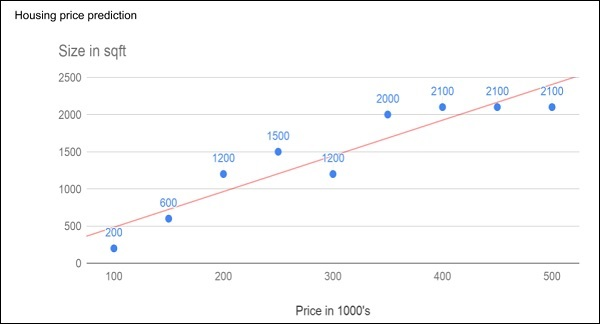After plotting various data points on the XY plot, we draw a best-fit line to do our predictions for any other house given its size. You will feed the known data to the machine and ask it to find the best fit line. Once the best fit line is found by the machine, you will test its suitability by feeding in a known house size, i.e. the Y-value in the above curve. The machine will now return the estimated X-value, i.e. the expected price of the house. The diagram can be extrapolated to find out the price of a house which is 3000 sq. ft. or even larger. This is called regression in statistics. Particularly, this kind of regression is called linear regression as the relationship between X & Y data points is linear.

In many cases, the relationship between the X & Y data points may not be a straight line, and it may be a curve with a complex equation. Your task would be now to find out the best fitting curve which can be extrapolated to predict the future values. One such application plot is shown in the figure below.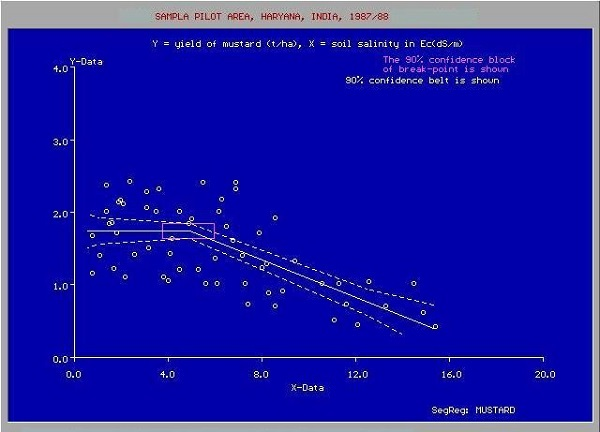Source:

You will use the statistical optimization techniques to find out the equation for the best fit curve here. And this is what exactly Machine Learning is about. You use known optimization techniques to find the best solution to your problem.

Next, let us look at the different categories of Machine Learning.

### Machine Learning – Categories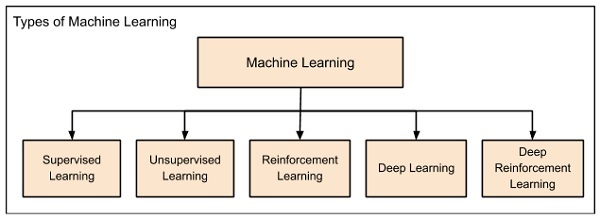Machine learning evolved from left to right as shown in the above diagram.

• Initially, researchers started out with Supervised Learning. This is the case of housing price prediction discussed earlier.
• This was followed by unsupervised learning, where the machine is made to learn on its own without any supervision.
• Scientists discovered further that it may be a good idea to reward the machine when it does the job the expected way and there came the Reinforcement Learning.
• Very soon, the data that is available these days has become so humongous that the conventional techniques developed so far failed to analyze the big data and provide us the predictions.
• Thus, came the deep learning where the human brain is simulated in the Artificial Neural Networks (ANN) created in our binary computers.
• The machine now learns on its own using the high computing power and huge memory resources that are available today.
• It is now observed that Deep Learning has solved many of the previously unsolvable problems.
• The technique is now further advanced by giving incentives to Deep Learning networks as awards and there finally comes Deep Reinforcement Learning.

Let us now study each of these categories in more detail.

### Supervised Learning

Supervised learning is analogous to training a child to walk. You will hold the child’s hand, show him how to take his foot forward, walk yourself for a demonstration and so on, until the child learns to walk on his own.

#### Regression

Similarly, in the case of supervised learning, you give concrete known examples to the computer. You say that for given feature value x1 the output is y1, for x2 it is y2, for x3 it is y3, and so on. Based on this data, you let the computer figure out an empirical relationship between x and y.

Once the machine is trained in this way with a sufficient number of data points, now you would ask the machine to predict Y for a given X. Assuming that you know the real value of Y for this given X, you will be able to deduce whether the machine’s prediction is correct.

Thus, you will test whether the machine has learned by using the known test data. Once you are satisfied that the machine is able to do the predictions with a desired level of accuracy (say 80 to 90%) you can stop further training the machine.

Now, you can safely use the machine to do the predictions on unknown data points, or ask the machine to predict Y for a given X for which you do not know the real value of Y. This training comes under the regression that we talked about earlier.

#### Classification

You may also use machine learning techniques for classification problems. In classification problems, you classify objects of similar nature into a single group. For example, in a set of 100 students say, you may like to group them into three groups based on their heights – short, medium and long. Measuring the height of each student, you will place them in a proper group.

Now, when a new student comes in, you will put him in an appropriate group by measuring his height. By following the principles in regression training, you will train the machine to classify a student based on his feature – the height. When the machine learns how the groups are formed, it will be able to classify any unknown new student correctly. Once again, you would use the test data to verify that the machine has learned your technique of classification before putting the developed model in production.

Supervised Learning is where the AI really began its journey. This technique was applied successfully in several cases. You have used this model while doing the hand-written recognition on your machine. Several algorithms have been developed for supervised learning. You will learn about them in the following chapters.

### Unsupervised Learning

In unsupervised learning, we do not specify a target variable to the machine, rather we ask machine “What can you tell me about X?”. More specifically, we may ask questions such as given a huge data set X, “What are the five best groups we can make out of X?” or “What features occur together most frequently in X?”. To arrive at the answers to such questions, you can understand that the number of data points that the machine would require to deduce a strategy would be very large. In case of supervised learning, the machine can be trained with even about few thousands of data points. However, in case of unsupervised learning, the number of data points that is reasonably accepted for learning starts in a few millions. These days, the data is generally abundantly available. The data ideally requires curating. However, the amount of data that is continuously flowing in a social area network, in most cases data curation is an impossible task.

The following figure shows the boundary between the yellow and red dots as determined by unsupervised machine learning. You can see it clearly that the machine would be able to determine the class of each of the black dots with a fairly good accuracy.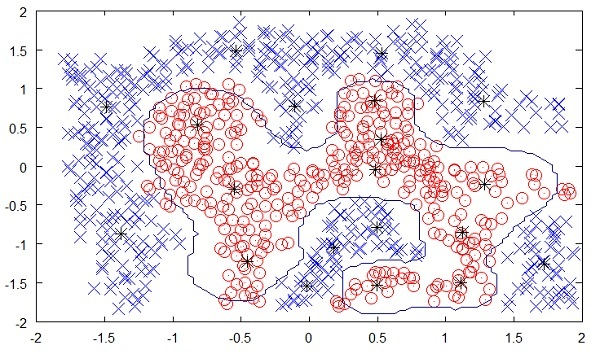Source:

https://chrisjmccormick.files.wordpress.com/2013/08/approx_decision_boun dary.png

The unsupervised learning has shown a great success in many modern AI applications, such as face detection, object detection, and so on.

### Reinforcement Learning

Consider training a pet dog, we train our pet to bring a ball to us. We throw the ball at a certain distance and ask the dog to fetch it back to us. Every time the dog does this right, we reward the dog. Slowly, the dog learns that doing the job rightly gives him a reward and then the dog starts doing the job right way every time in future. Exactly, this concept is applied in “Reinforcement” type of learning. The technique was initially developed for machines to play games. The machine is given an algorithm to analyze all possible moves at each stage of the game. The machine may select one of the moves at random. If the move is right, the machine is rewarded, otherwise it may be penalized. Slowly, the machine will start differentiating between right and wrong moves and after several iterations would learn to solve the game puzzle with a better accuracy. The accuracy of winning the game would improve as the machine plays more and more games.

The entire process may be depicted in the following diagram −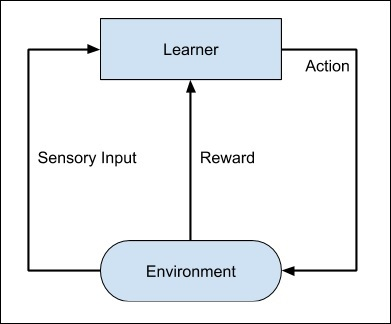This technique of machine learning differs from the supervised learning in that you need not supply the labelled input/output pairs. The focus is on finding the balance between exploring the new solutions versus exploiting the learned solutions.

### Deep Learning

The deep learning is a model based on Artificial Neural Networks (ANN), more specifically Convolutional Neural Networks (CNN)s. There are several architectures used in deep learning such as deep neural networks, deep belief networks, recurrent neural networks, and convolutional neural networks.

These networks have been successfully applied in solving the problems of computer vision, speech recognition, natural language processing, bioinformatics, drug design, medical image analysis, and games. There are several other fields in which deep learning is proactively applied. The deep learning requires huge processing power and humongous data, which is generally easily available these days.

We will talk about deep learning more in detail in the coming chapters.

### Deep Reinforcement Learning

The Deep Reinforcement Learning (DRL) combines the techniques of both deep and reinforcement learning. The reinforcement learning algorithms like Q-learning are now combined with deep learning to create a powerful DRL model. The technique has been with a great success in the fields of robotics, video games, finance and healthcare. Many previously unsolvable problems are now solved by creating DRL models. There is lots of research going on in this area and this is very actively pursued by the industries.

So far, you have got a brief introduction to various machine learning models, now let us explore slightly deeper into various algorithms that are available under these models.

### Machine Learning – Supervised

Supervised learning is one of the important models of learning involved in training machines. This chapter talks in detail about the same.

### Algorithms for Supervised Learning

There are several algorithms available for supervised learning. Some of the widely used algorithms of supervised learning are as shown below −

• k-Nearest Neighbours
• Decision Trees
• Naive Bayes
• Logistic Regression
• Support Vector Machines

As we move ahead in this chapter, let us discuss in detail about each of the algorithms.

### k-Nearest Neighbours

The k-Nearest Neighbours, which is simply called kNN is a statistical technique that can be used for solving for classification and regression problems. Let us discuss the case of classifying an unknown object using kNN. Consider the distribution of objects as shown in the image given below −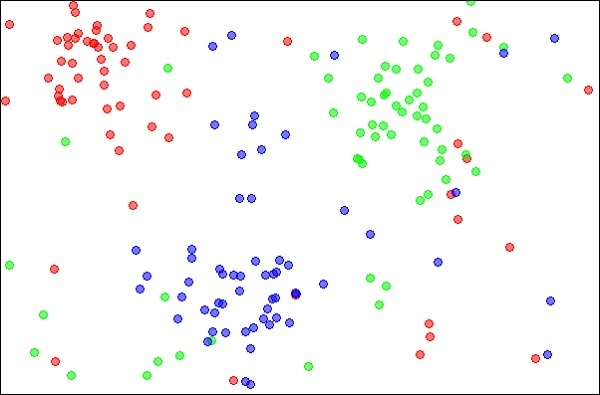Source:

https://en.wikipedia.org/wiki/K-nearest_neighbors_algorithm

The diagram shows three types of objects, marked in red, blue and green colors. When you run the kNN classifier on the above dataset, the boundaries for each type of object will be marked as shown below −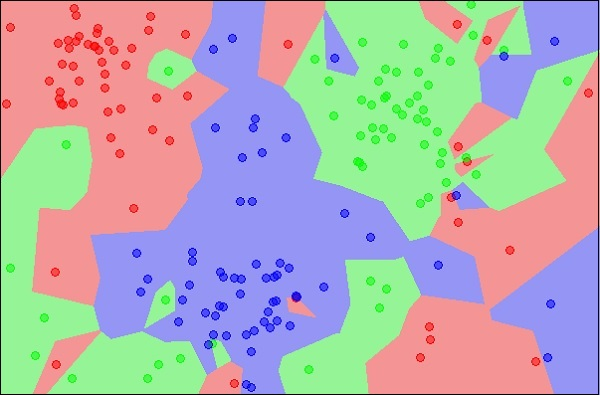Source:

https://en.wikipedia.org/wiki/K-nearest_neighbors_algorithm

Now, consider a new unknown object that you want to classify as red, green or blue. This is depicted in the figure below.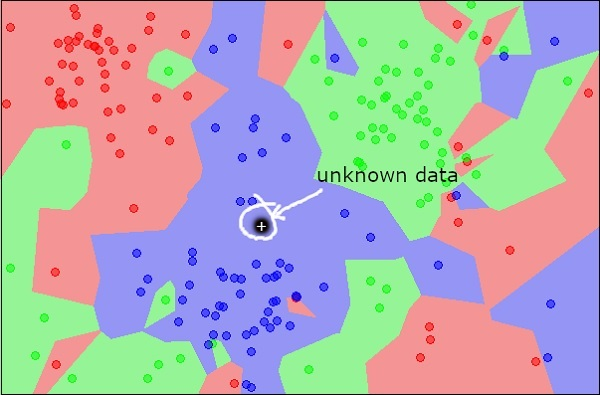As you see it visually, the unknown data point belongs to a class of blue objects. Mathematically, this can be concluded by measuring the distance of this unknown point with every other point in the data set. When you do so, you will know that most of its neighbours are of blue color. The average distance to red and green objects would be definitely more than the average distance to blue objects. Thus, this unknown object can be classified as belonging to blue class.

The kNN algorithm can also be used for regression problems. The kNN algorithm is available as ready-to-use in most of the ML libraries.

### Decision Trees

A simple decision tree in a flowchart format is shown below −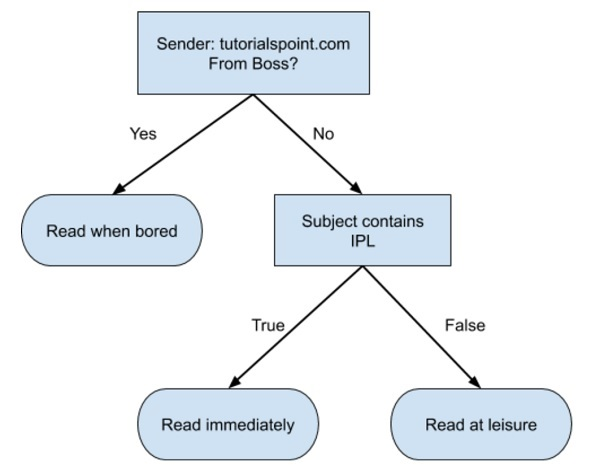You would write a code to classify your input data based on this flowchart. The flowchart is self-explanatory and trivial. In this scenario, you are trying to classify an incoming email to decide when to read it.

In reality, the decision trees can be large and complex. There are several algorithms available to create and traverse these trees. As a Machine Learning enthusiast, you need to understand and master these techniques of creating and traversing decision trees.

### Naive Bayes

Naive Bayes is used for creating classifiers. Suppose you want to sort out (classify) fruits of different kinds from a fruit basket. You may use features such as color, size and shape of a fruit, For example, any fruit that is red in color, is round in shape and is about 10 cm in diameter may be considered as Apple. So to train the model, you would use these features and test the probability that a given feature matches the desired constraints. The probabilities of different features are then combined to arrive at a probability that a given fruit is an Apple. Naive Bayes generally requires a small number of training data for classification.

### Logistic Regression

Look at the following diagram. It shows the distribution of data points in XY plane.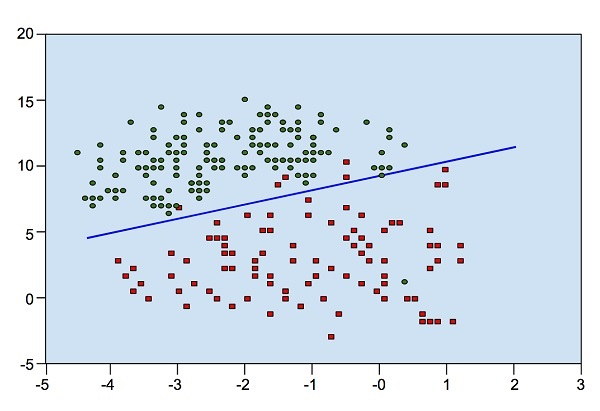From the diagram, we can visually inspect the separation of red dots from green dots. You may draw a boundary line to separate out these dots. Now, to classify a new data point, you will just need to determine on which side of the line the point lies.

### Support Vector Machines

Look at the following distribution of data. Here the three classes of data cannot be linearly separated. The boundary curves are non-linear. In such a case, finding the equation of the curve becomes a complex job.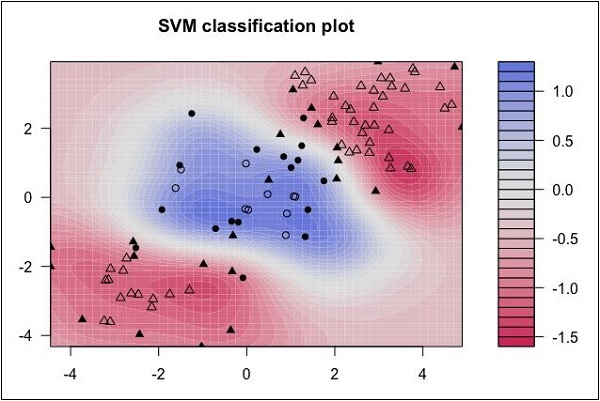Source: http://uc-r.github.io/svm

The Support Vector Machines (SVM) comes handy in determining the separation boundaries in such situations.

### Machine Learning – Scikit-learn Algorithm

Fortunately, most of the time you do not have to code the algorithms mentioned in the previous lesson. There are many standard libraries which provide the ready-to-use implementation of these algorithms. One such toolkit that is popularly used is scikit-learn. The figure below illustrates the kind of algorithms which are available for your use in this library.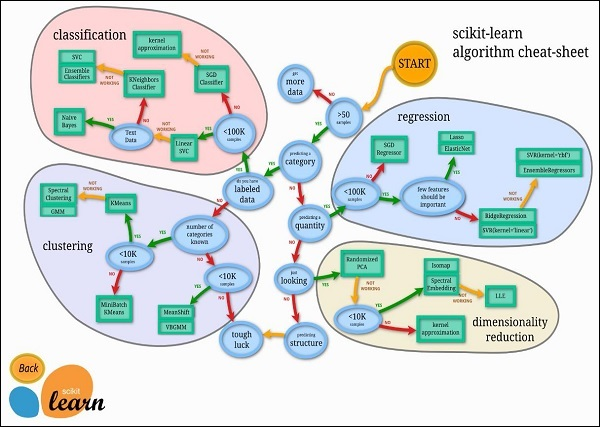Source: https://scikit-learn.org/stable/tutorial/machine_learning_map/index.html

The use of these algorithms is trivial and since these are well and field tested, you can safely use them in your AI applications. Most of these libraries are free to use even for commercial purposes.

### Machine Learning – Unsupervised

So far what you have seen is making the machine learn to find out the solution to our target. In regression, we train the machine to predict a future value. In classification, we train the machine to classify an unknown object in one of the categories defined by us. In short, we have been training machines so that it can predict Y for our data X. Given a huge data set and not estimating the categories, it would be difficult for us to train the machine using supervised learning. What if the machine can look up and analyze the big data running into several Gigabytes and Terabytes and tell us that this data contains so many distinct categories?

As an example, consider the voter’s data. By considering some inputs from each voter (these are called features in AI terminology), let the machine predict that there are so many voters who would vote for X political party and so many would vote for Y, and so on. Thus, in general, we are asking the machine given a huge set of data points X, “What can you tell me about X?”. Or it may be a question like “What are the five best groups we can make out of X?”. Or it could be even like “What three features occur together most frequently in X?”.

This is exactly the Unsupervised Learning is all about.

### Algorithms for Unsupervised Learning

Let us now discuss one of the widely used algorithms for classification in unsupervised machine learning.

#### k-means clustering

The 2000 and 2004 Presidential elections in the United States were close — very close. The largest percentage of the popular vote that any candidate received was 50.7% and the lowest was 47.9%. If a percentage of the voters were to have switched sides, the outcome of the election would have been different. There are small groups of voters who, when properly appealed to, will switch sides. These groups may not be huge, but with such close races, they may be big enough to change the outcome of the election. How do you find these groups of people? How do you appeal to them with a limited budget? The answer is clustering.

Let us understand how it is done.

• First, you collect information on people either with or without their consent: any sort of information that might give some clue about what is important to them and what will influence how they vote.
• Then you put this information into some sort of clustering algorithm.
• Next, for each cluster (it would be smart to choose the largest one first) you craft a message that will appeal to these voters.
• Finally, you deliver the campaign and measure to see if it’s working.

Clustering is a type of unsupervised learning that automatically forms clusters of similar things. It is like automatic classification. You can cluster almost anything, and the more similar the items are in the cluster, the better the clusters are. In this chapter, we are going to study one type of clustering algorithm called k-means. It is called k-means because it finds ‘k’ unique clusters, and the center of each cluster is the mean of the values in that cluster.

#### Cluster Identification

Cluster identification tells an algorithm, “Here’s some data. Now group similar things together and tell me about those groups.” The key difference from classification is that in classification you know what you are looking for. While that is not the case in clustering.

Clustering is sometimes called unsupervised classification because it produces the same result as classification does but without having predefined classes.

Now, we are comfortable with both supervised and unsupervised learning. To understand the rest of the machine learning categories, we must first understand Artificial Neural Networks (ANN), which we will learn in the next chapter.

### Machine Learning – Artificial Neural Networks

The idea of artificial neural networks was derived from the neural networks in the human brain. The human brain is really complex. Carefully studying the brain, the scientists and engineers came up with an architecture that could fit in our digital world of binary computers. One such typical architecture is shown in the diagram below −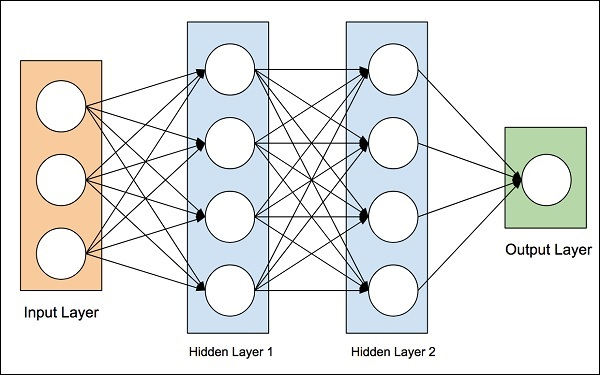There is an input layer which has many sensors to collect data from the outside world. On the right hand side, we have an output layer that gives us the result predicted by the network. In between these two, several layers are hidden. Each additional layer adds further complexity in training the network, but would provide better results in most of the situations. There are several types of architectures designed which we will discuss now.

### ANN Architectures

The diagram below shows several ANN architectures developed over a period of time and are in practice today.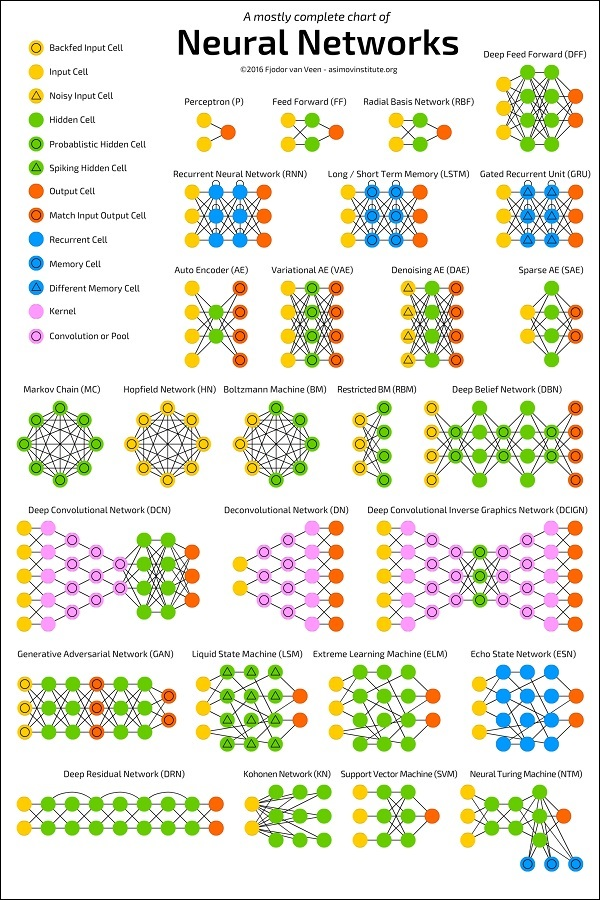Source:

https://towardsdatascience.com/the-mostly-complete-chart-of-neural-networks-explained-3fb6f2367464

Each architecture is developed for a specific type of application. Thus, when you use a neural network for your machine learning application, you will have to use either one of the existing architecture or design your own. The type of application that you finally decide upon depends on your application needs. There is no single guideline that tells you to use a specific network architecture.

### Machine Learning – Deep Learning

Deep Learning uses ANN. First we will look at a few deep learning applications that will give you an idea of its power.

#### Applications

Deep Learning has shown a lot of success in several areas of machine learning applications.

Self-driving Cars − The autonomous self-driving cars use deep learning techniques. They generally adapt to the ever changing traffic situations and get better and better at driving over a period of time.

Speech Recognition − Another interesting application of Deep Learning is speech recognition. All of us use several mobile apps today that are capable of recognizing our speech. Apple’s Siri, Amazon’s Alexa, Microsoft’s Cortena and Google’s Assistant – all these use deep learning techniques.

Mobile Apps − We use several web-based and mobile apps for organizing our photos. Face detection, face ID, face tagging, identifying objects in an image – all these use deep learning.

### Untapped Opportunities of Deep Learning

After looking at the great success deep learning applications have achieved in many domains, people started exploring other domains where machine learning was not so far applied. There are several domains in which deep learning techniques are successfully applied and there are many other domains which can be exploited. Some of these are discussed here.

• Agriculture is one such industry where people can apply deep learning techniques to improve the crop yield.
• Consumer finance is another area where machine learning can greatly help in providing early detection on frauds and analyzing customer’s ability to pay.
• Deep learning techniques are also applied to the field of medicine to create new drugs and provide a personalized prescription to a patient.

The possibilities are endless and one has to keep watching as the new ideas and developments pop up frequently.

### What is Required for Achieving More Using Deep Learning

To use deep learning, supercomputing power is a mandatory requirement. You need both memory as well as the CPU to develop deep learning models. Fortunately, today we have an easy availability of HPC – High Performance Computing. Due to this, the development of the deep learning applications that we mentioned above became a reality today and in the future too we can see the applications in those untapped areas that we discussed earlier.

Now, we will look at some of the limitations of deep learning that we must consider before using it in our machine learning application.

Some of the important points that you need to consider before using deep learning are listed below −

• Black Box approach
• Duration of Development
• Amount of Data
• Computationally Expensive

We will now study each one of these limitations in detail.

#### Black Box approach

An ANN is like a blackbox. You give it a certain input and it will provide you a specific output. The following diagram shows you one such application where you feed an animal image to a neural network and it tells you that the image is of a dog.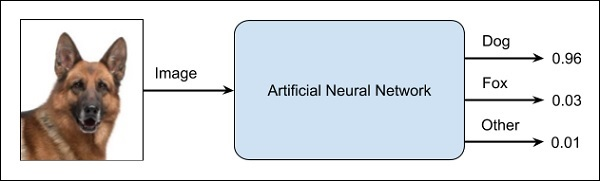Why this is called a black-box approach is that you do not know why the network came up with a certain result. You do not know how the network concluded that it is a dog? Now consider a banking application where the bank wants to decide the creditworthiness of a client. The network will definitely provide you an answer to this question. However, will you be able to justify it to a client? Banks need to explain it to their customers why the loan is not sanctioned?

#### Duration of Development

The process of training a neural network is depicted in the diagram below −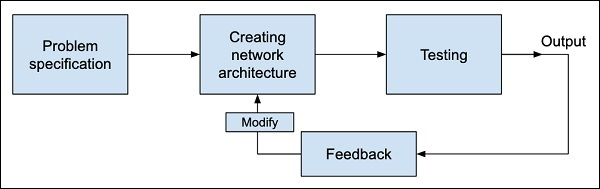You first define the problem that you want to solve, create a specification for it, decide on the input features, design a network, deploy it and test the output. If the output is not as expected, take this as a feedback to restructure your network. This is an iterative process and may require several iterations until the time network is fully trained to produce desired outputs.

#### Amount of Data

The deep learning networks usually require a huge amount of data for training, while the traditional machine learning algorithms can be used with a great success even with just a few thousands of data points. Fortunately, the data abundance is growing at 40% per year and CPU processing power is growing at 20% per year as seen in the diagram given below −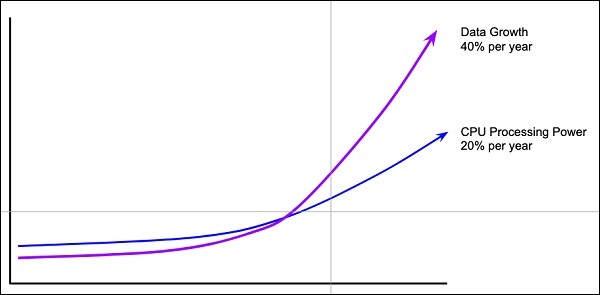#### Computationally Expensive

Training a neural network requires several times more computational power than the one required in running traditional algorithms. Successful training of deep Neural Networks may require several weeks of training time.

In contrast to this, traditional machine learning algorithms take only a few minutes/hours to train. Also, the amount of computational power needed for training deep neural network heavily depends on the size of your data and how deep and complex the network is?

After having an overview of what Machine Learning is, its capabilities, limitations, and applications, let us now dive into learning “Machine Learning”.

### Machine Learning – Skills

Machine Learning has a very large width and requires skills across several domains. The skills that you need to acquire for becoming an expert in Machine Learning are listed below −

• Statistics
• Probability Theories
• Calculus
• Optimization techniques
• Visualization

### Necessity of Various Skills of Machine Learning

To give you a brief idea of what skills you need to acquire, let us discuss some examples −

#### Mathematical Notation

Most of the machine learning algorithms are heavily based on mathematics. The level of mathematics that you need to know is probably just a beginner level. What is important is that you should be able to read the notation that mathematicians use in their equations. For example – if you are able to read the notation and comprehend what it means, you are ready for learning machine learning. If not, you may need to brush up your mathematics knowledge.

$$f_{AN}(net-\theta)=\begin{cases}\gamma & if\:net-\theta \geq \epsilon\\net-\theta & if – \epsilon< net-\theta <\epsilon\\ -\gamma & if\:net-\theta\leq- \epsilon\end{cases}$$

$$\displaystyle\\\max\limits_{\alpha}\begin{bmatrix}\displaystyle\sum\limits_{i=1}^m \alpha-\frac{1}{2}\displaystyle\sum\limits_{i,j=1}^m label^\left(\begin{array}{c}i\\ \end{array}\right)\cdot\:label^\left(\begin{array}{c}j\\ \end{array}\right)\cdot\:a_{i}\cdot\:a_{j}\langle x^\left(\begin{array}{c}i\\ \end{array}\right),x^\left(\begin{array}{c}j\\ \end{array}\right)\rangle \end{bmatrix}$$

$$f_{AN}(net-\theta)=\left(\frac{e^{\lambda(net-\theta)}-e^{-\lambda(net-\theta)}}{e^{\lambda(net-\theta)}+e^{-\lambda(net-\theta)}}\right)\;$$

#### Probability Theory

Here is an example to test your current knowledge of probability theory: Classifying with conditional probabilities.

$$p(c_{i}|x,y)\;=\frac{p(x,y|c_{i})\;p(c_{i})\;}{p(x,y)\;}$$

With these definitions, we can define the Bayesian classification rule −

• If P(c1|x, y) > P(c2|x, y) , the class is c1 .
• If P(c1|x, y) < P(c2|x, y) , the class is c2 .

#### Optimization Problem

Here is an optimization function

$$\displaystyle\\\max\limits_{\alpha}\begin{bmatrix}\displaystyle\sum\limits_{i=1}^m \alpha-\frac{1}{2}\displaystyle\sum\limits_{i,j=1}^m label^\left(\begin{array}{c}i\\ \end{array}\right)\cdot\:label^\left(\begin{array}{c}j\\ \end{array}\right)\cdot\:a_{i}\cdot\:a_{j}\langle x^\left(\begin{array}{c}i\\ \end{array}\right),x^\left(\begin{array}{c}j\\ \end{array}\right)\rangle \end{bmatrix}$$

Subject to the following constraints −

$$\alpha\geq0,and\:\displaystyle\sum\limits_{i-1}^m \alpha_{i}\cdot\:label^\left(\begin{array}{c}i\\ \end{array}\right)=0$$

If you can read and understand the above, you are all set.

#### Visualization

In many cases, you will need to understand the various types of visualization plots to understand your data distribution and interpret the results of the algorithm’s output.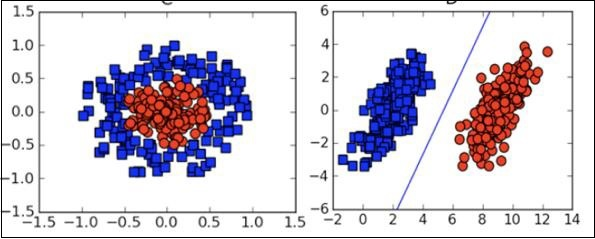Besides the above theoretical aspects of machine learning, you need good programming skills to code those algorithms.

So what does it take to implement ML? Let us look into this in the next chapter.

### Machine Learning – Implementing

To develop ML applications, you will have to decide on the platform, the IDE and the language for development. There are several choices available. Most of these would meet your requirements easily as all of them provide the implementation of AI algorithms discussed so far.

If you are developing the ML algorithm on your own, the following aspects need to be understood carefully −

The language of your choice − this essentially is your proficiency in one of the languages supported in ML development.

The IDE that you use − This would depend on your familiarity with the existing IDEs and your comfort level.

Development platform − There are several platforms available for development and deployment. Most of these are free-to-use. In some cases, you may have to incur a license fee beyond a certain amount of usage. Here is a brief list of choice of languages, IDEs and platforms for your ready reference.

### Language Choice

Here is a list of languages that support ML development −

• Python
• R
• Matlab
• Octave
• Julia
• C++
• C

This list is not essentially comprehensive; however, it covers many popular languages used in machine learning development. Depending upon your comfort level, select a language for the development, develop your models and test.

### IDEs

Here is a list of IDEs which support ML development −

• R Studio
• Pycharm
• iPython/Jupyter Notebook
• Julia
• Spyder
• Anaconda
• Rodeo

The above list is not essentially comprehensive. Each one has its own merits and demerits. The reader is encouraged to try out these different IDEs before narrowing down to a single one.

### Platforms

Here is a list of platforms on which ML applications can be deployed −

• IBM
• Microsoft Azure
• Amazon
• Mlflow

Once again this list is not exhaustive. The reader is encouraged to sign-up for the abovementioned services and try them out themselves.

### Machine Learning – Conclusion

This tutorial has introduced you to Machine Learning. Now, you know that Machine Learning is a technique of training machines to perform the activities a human brain can do, albeit bit faster and better than an average human-being. Today we have seen that the machines can beat human champions in games such as Chess, AlphaGO, which are considered very complex. You have seen that machines can be trained to perform human activities in several areas and can aid humans in living better lives.

Machine Learning can be a Supervised or Unsupervised. If you have lesser amount of data and clearly labelled data for training, opt for Supervised Learning. Unsupervised Learning would generally give better performance and results for large data sets. If you have a huge data set easily available, go for deep learning techniques. You also have learned Reinforcement Learning and Deep Reinforcement Learning. You now know what Neural Networks are, their applications and limitations.

Finally, when it comes to the development of machine learning models of your own, you looked at the choices of various development languages, IDEs and Platforms. Next thing that you need to do is start learning and practicing each machine learning technique. The subject is vast, it means that there is width, but if you consider the depth, each topic can be learned in a few hours. Each topic is independent of each other. You need to take into consideration one topic at a time, learn it, practice it and implement the algorithm/s in it using a language choice of yours. This is the best way to start studying Machine Learning. Practicing one topic at a time, very soon you would acquire the width that is eventually required of a Machine Learning expert.

Good Luck!

Related Articles